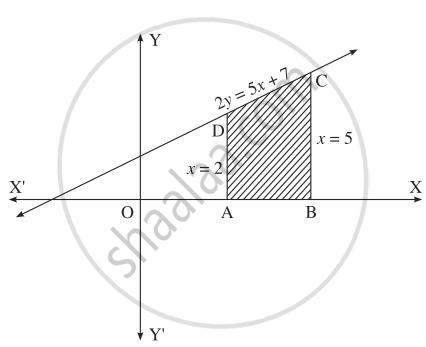# Solve the following : Find the area of the region bounded by the straight line 2y = 5x + 7, X-axis and x = 2, x = 5. - Mathematics and Statistics

Sum

Solve the following :

Find the area of the region bounded by the straight line 2y = 5x + 7, X-axis and x = 2, x = 5.

#### Solution

The equation of the line is 2y = 5x+ 7,

i.e., y = (5)/(2)x + (7)/(2)
Required area = area of the region ABCDA

= area under the line y = 5)/(2)x + (7)/(2) between x = 2 and x = 5= int_2^5 (5/2x + 7/2)*dx

= (5)/(2)* int_2^5x*dx + (7)/(2) int_2^5 1*dx

= (5)/(2)[x^2/2]_2^5 + (7)/(2)[x]_2^5

= (5)/(2)[25/2 - 4/2] + (7)/(2)[5 - 2]

= (5)/(2) xx (21)/(2) + (21)/(2)

= (105)/(4) + (42)/(4)

= (147)/(4)"sq units".

Concept: Area Bounded by the Curve, Axis and Line
Is there an error in this question or solution?

#### APPEARS IN

Balbharati Mathematics and Statistics 2 (Arts and Science) 12th Standard HSC Maharashtra State Board
Chapter 5 Application of Definite Integration
Miscellaneous Exercise 5 | Q 2.09 | Page 190